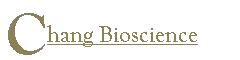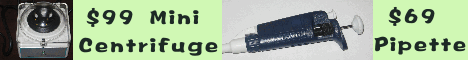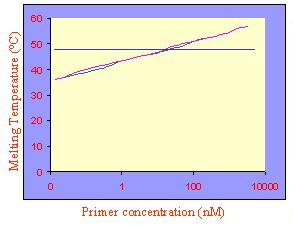Online | Download | Store | Contact | About

How to calculate melting temperature for degenerate primers?

Primo Pro Online
Primo Multiplex Online --- multiplex PCR primer design
Primo Degenerate Online --- degenerate PCR primer design
Primo MSP Online --- methylation specific PCR primer design

Common sense tells us that higher the primer concentration, better the chance for the primer to bind to the template, thus higher the melting temperature. Most primer design programs or melting temperature formulae don't take into account this fact. For example, the widely-used formula based on counting the number of AT or CG (2 °C for each A and T, 4 °C for each C and G) does not have a correction for the primer concentration.

 For non-degenerate primers, the correction due to primer concentration is a small factor in most cases. It is however not negligible for degenerate primers, since the effective primer concentration should be divided by the fold of degeneracy. Most primer design programs failed to notice that the melting temperature is dependent on the fold of degeneracy and will do poorly in designing degenerate primers because of the wrong melting temperature predictions. As the primer concentration decreases, the melting temperature decreases (Figure 1). Over a wide range of primer concentration, the melting temperature decreases 1 degree for each 2 fold decrease in the primer concentration (Figure 1). If the degeneracy for a primer is 1000 fold, the melting temperature should be approximately 10 degree lower compared to that calculated without the primer concentration correction (i.e., 2 °C for each A and T, 4 °C for each C and G). If you use Primo and select the Nearest Neighbor formula, there is no need to correct for the effective primer concentration for degenerate primers---it has been taken care of. Users are strongly suggested to use the Nearest Neighbor formula in designing degenerate primers or if the template is degenerate.Figure 1. The effect of primer concentration on melting temperature. The melting temperature of two 16-mers (8 A or T and 8 C or G) are shown. Melting temperature is calculated using the Nearest Neighbor model.

Copyright © 2002-2004 Chang Bioscience, Inc. All rights reserved.